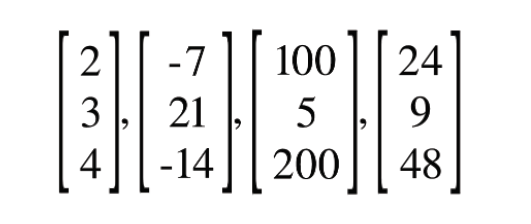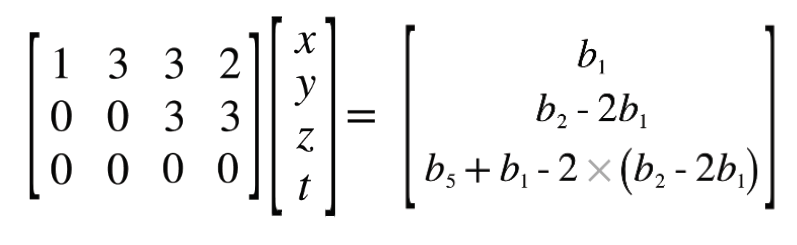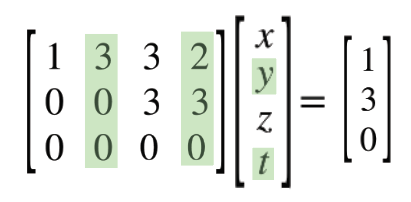# Linear Algebra 5: Solving Ax = b in non-invertible, non-square matrices

This is a continuation of my Linear Algebra series, which should be viewed as an extra resource while going along with Gilbert Strang’s class 18.06 on OCW. This can be closely matched to Lecture 8 in his series.

Here, we continue our discussion about solving linear systems with elimination. In my Gaussian Elimination series, we explored how square, invertible matrices can be solved by method of elimination and row exchanges — but we never delved into solving rectangular, non-invertible systems.

In the last lesson, we explored how non-square systems can be solved by using Gaussian elimination. Specifically, we solved systems in the format Ax = 0, and discovered the null space vectors x that made x = 0.

The biggest difference between Ax = b and Ax = 0 is now that our right hand side is nonzero, we have to carry out the operations on both sides. This leads us to our first topic, which is the condition for solvability.

## Conditions for Solvability

All of these examples will deal with rectangular, non-invertible matrices. If you have a square and invertible matrix (which is often the case) use the more straightforward Gaussian Elimination.

The conditions for solvability are how we recognize if a certain b in Ax = b is a solution that actually is possible. This will become abundantly clear with an example. Remember that now, when going through elimination with matrices that we expect to be dependent, we won’t stop at pivots in the zero place. We will just keep going to the next column.

Our goal is to find out what the answer b has to look like for a problem to be solvable.

Okay, first example.

Rows one and three are multiples of each other, and will cancel out to a row of zeros after elimination. After using the first row to eliminate the first and second rows, we get:

But now that we have nonzero numbers on our right hand side, we have to have done these same steps of elimination on the right hand side.

What did we do? To eliminate the second row, we multiplied the first row by negative one and subtracted from the bottom row (or, you could think, we added the first row). Then, we multiplied the first row by 2 and subtracted from the final row. So now, with our variables b, what does this look like?

Because of the equality, we have to do the same row operations on the rows of b. Thus, after this, we can get an idea of what our b’s look like.

Solvability conditions come up when the left hand side is 0. If we were to write this out as a system of equations, we would get:

The first two equations don’t give us much information or restrictions on b, as they are dependent on what values we end up giving to our variables x, y, z and t.

The last equation, on the other hand, gives us a definite restriction on b. For Ax = b to be true, b3 – 2b1 must = 0. Rearranging this, we get the restriction on b that:

Now, we are limited in the different b3’s we can put in our matrix. A few examples of b vectors that do satisfy this restriction include:In all of these, b3 is 2 times b1.

This can give us a quick impression if an Ax = b is possible or not, or, when making an equation, can give us an idea of the possible answers we can have.

This gives us two different ways to understand this specific Ax = b.

• Using our knowledge of Columnspace, we know that b must be a part of the column space of A. In other words, bC(A)
• Our new understanding — b must satisfy all restrictions on it.

Now that we know this, let’s actually solve a problem.

## Solving for the Complete Solution to Ax = b

I’m taking this example directly from page 91 of Gilbert Strang’s textbook Linear Algebra and it’s Applications, 4th edition.

Let’s take this system:

To solve this, we first need to find an answer b that satisfies any restrictions we may have. To find these restrictions (and simultaneously, take the A to U), use gaussian elimination to eliminate the left and right hand sides.

We multiplied the first row by 2 and subtracted from the second row. Then we multiplied the first row by -1 and subtracted from the third row (added). We reflected these same changes on the right hand side.

Now, we must eliminate the final row with our second row.That b5 should be a b3 but I’m too lazy to change it

Here, we subtract two times the second row from the third row. We need to remember to do this on the right hand side. Here, it gets a little messy, since we’re building on past elimination steps, but we can still do it.

Again, our condition for solvability is in the third row. If we simplify this, we get our condition for solvability.

Now that we have our condition for solvability, we can pick an answer b that works with it. Why don’t we pick (1, 5, 5)? You can check yourself that this works.

Just a reminder that once we find our b to replace it in the original Ax = b equation, with A on the left hand side and not U.

Now, we undergo the same process of elimination, just this time with concrete numbers, and as predicted, it satisfies the restraint we put on it.

## A note on the Complete Solution

Now, looking at this, there are a variety of solutions. But the critical thing to point out is that our final answer will have to include all of the null space answers as well.

Let me explain. Since this matrix A is not independent, there will surely be at least one vector x in the null space. This x solves for Ax = 0.

Thus, if we find a particular solution to this, some x that solves Ax = b, we must also add all our null space vectors for a full answer. Since all the null space vectors make Ax = 0, our full answer should include A(x_null + x_particular) = b, since adding the null space does nothing to b, since Ax_null = 0.

If this doesn’t make sense, let’s keep going.

Let’s first find a particular solution to this equation. This is an x that directly solves for Ax = b.

## Finding a Particular Solution

We are aiming to find a particular solution, some value of x, y, z and t that solves for our answer (1, 3, 0). Since we only have two complete equations, we can only find out two of these variables. The other two we will have to set to constants.

Again, similar to solving for the null space, the variables we will set as constants will be the free variables. These are the variables associated with the free columns, which are the columns that don’t contain pivots.Highlighted are the free columns and variables.

We have a double infinity of answers once again, since we have two variables that we can set as any constants. Thus, we have an infinite amount of particular answers that solve for (1, 3, 0). So, if we can choose any constant for y or t, we can choose the easiest, which is 0, for both.

After doing this, our linear combination and system of equations look like:

Thus, one of (our many possible) particular solutions has been made. As I said, this is not the complete answer. We can add any vector from the null space (and any of their multiples) to this particular answer and still get Ax = b, since all of these null space vectors make Ax = 0.

Thus, we must also solve for the null space vectors. I already covered how to calculate null space vectors in a very similar matrix, so I won’t walk through it this time, and just give you the answers to the null space vectors.

So, now we can add this combination to our single particular answer. It is important to note that although the null space vectors are a combination, we cannot have a multiple in front of our particular answer — since our right hand side is not zero, we can’t simply multiply each x, y, z, t and get an equivalent answer. So, our final answer is:

Graphically, what does this answer look like? Well, it’s not a subspace. You can think of it as an almost augmented null space — the null space but with every point shifted by the vector xp. Thus, we no longer run through the origin, but xp.

And that’s our complete solution to Ax = b.

There’s just one more thing. Similar to our null space article, we can employ the reduced row-echelon form to make the answer even simpler.

## Finding the Particular Solution Immediately with RREF

Let’s rewind back to the point where we had the system Ux = c. We can further reduce this U into R, and take our c to d. How would we do this?

Well, just like last article, we would use Gauss-Jordan elimination to eliminate upwards after making all pivots into one. The only added difficulty here is we must do these same operations to the right side as well, since the right side is now nonzero.

The only pivot we need to turn into zero is in the second row. Divide each item in the row, and the corresponding item in b, to do this.

We can then multiply this second row by three to eliminate the three above the pivot in the first row. Then we also do the same on the right hand side.

Now, our matrix system is in Rx = b. Now, we can directly extract our particular solution xp. The answer is hidden in plain sight.

Since we turn our free variables y and t into into 0. Thinking in linear combination form, these zeros eliminate the two free columns 2 and 4.

Then, we’re left with the identity, multiplied by x = d.

So, to solve for this, our particular solution IS d. Thus, when looking for the particular solution, we can just convert our matrix A into R, and take the right hand side d as our particular solution.

--

--